# Energy Stored in a Capacitor

Posted on

While capacitor is connected across a battery, charges come from the battery and energy stored in the capacitor plates. But this process of energy storing is step by step only. At the very beginning, capacitor does not have any charge or potential. i.e. V = 0 volts and q = 0 C. And also the energy stored in a capacitor can be calculated from the equivalent expressions: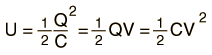Now at the time of switching, full battery voltage will fall across the capacitor. A positive charge (q) will come to the positive plate of the capacitor, but there is no work done for this first charge (q) to come to the positive plate of the capacitor from the battery.

# Energy Stored in a Capacitor

It is because of the capacitor does not have own voltage across its plates, rather the initial voltage is due to the battery. First charge grows little amount of voltage across the capacitor plates, and then second positive charge will come to the positive plate of the capacitor, but gets repealed by the first charge. As the battery voltage is more than the capacitor voltage then this second charge will be stored in the positive plate.

At that condition a little amount of work is to be done to store second charge in the capacitor. Again for the third charge, same phenomenon will appear. Gradually charges will come to be stored in the capacitor against pre-stored charges and their little amount of work done grows up.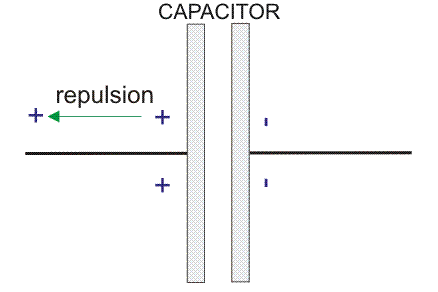## Two Plates of The Capacitor

It can’t be said that the capacitor voltage is fixed. It is because of the capacitor voltage is not fixed from the very beginning. It will be at its maximum limit when potency of capacitor will be equal to that of the battery.

As storage of charges increases, the voltage of the capacitor increases and also energy of the capacitor increases.

So at that point of discussion the energy equation for the capacitor can’t be written as energy (E) = V.q

As the voltage increases the electric field (E) inside the capacitor dielectric increases gradually but in opposite direction i.e. from positive plate to negative plate.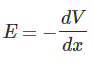Here dx is the distance between two plates of the capacitor.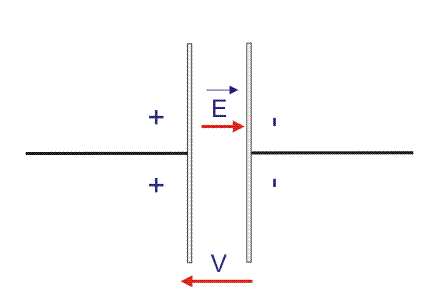## Calculate The Energy of The Capacitor

Charge will flow from battery to the capacitor plate until the capacitor gains as same potency as the battery. So, we have to calculate the energy of the capacitor from the very begging to the last moment of charge getting full.

Suppose, a small charge q is stored in the positive plate of the capacitor with respect to the battery voltage V and a small work done is dW.

Then considering the total charging time, we can write that,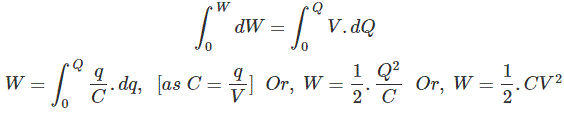Now we go for the energy loss during the charging time of a capacitor by a battery.

As the battery is in the fixed voltage the energy loss by the battery always follows the equation, W = V.q, this equation is not applicable for the capacitor as it does not have the fixed voltage from the very beginning of charging by the battery.

Now, the charge collected by the capacitor from the battery is: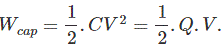Now charge lost by the battery is: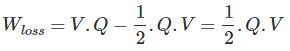This half energy from total amount of energy goes to the capacitor and rest half of energy automatically gets lost from the battery and it should be kept in mind always.

### Energy Stored in a Capacitor Conclusion

After going through the above calculate the energy of the capacitor we can now establish energy stored in a capacitor. I hope you enjoy when reading this article, thank you.